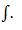Goseeko blog# What is integration?

IntroductionIntegration is the reverse process of differentiation. in other words It is also called anti-differentiation.

Integration calculus has its own application in economics, Engineering, Physics, Chemistry, business, commerce, etc.

The integral of a function is denoted by the signLet the function is y = f(x), So that its derivative is-

Then

Where c is the arbitrary constant.

For example,

A function,

Then, its derivative-

Or

Then

Here c is an arbitrary constant.

Some fundamental integrals-

## Methods of integration

Simple integration-

1.     When the function is an algebraic function-

Some standard form are-

The integration of  x^n will be as follows-

Example: Find the integral of-

Sol.

We know that-

Then

Example: Find the integral

Sol.

We know that-

Then

Example: Evaluate-

Sol.

## By substitution

Example: Evaluate the following integral-

Sol.

Let us suppose,

Then

Or

Substituting –

Logarithmic function-

Example: Evaluate the following integral-

Sol.

Let us suppose-

Now

## Integral of exponential function

Example: Evaluate-

Sol.

Let,

Now substituting-

Integration of product of two functions-

Suppose we have two function say- f(x) and g(x), then

The integral of product of these two functions is-

Note-

We chose the first function as method of ILATE-

Which is-

I – Inverse trigonometric function

L – Log function

A – Algebraic function

T- Trigonometric function

E- Exponential function

Example: Evaluate-

Sol.

Here according to ILATE,

First function = log x

Second function = x^n

We know that-

Then

On solving, we get-